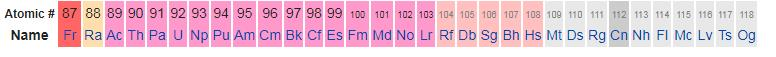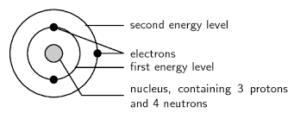Courses

# Test: Nomenclature Of Elements

## 20 Questions MCQ Test Chemistry for JEE | Test: Nomenclature Of Elements

Description
This mock test of Test: Nomenclature Of Elements for JEE helps you for every JEE entrance exam. This contains 20 Multiple Choice Questions for JEE Test: Nomenclature Of Elements (mcq) to study with solutions a complete question bank. The solved questions answers in this Test: Nomenclature Of Elements quiz give you a good mix of easy questions and tough questions. JEE students definitely take this Test: Nomenclature Of Elements exercise for a better result in the exam. You can find other Test: Nomenclature Of Elements extra questions, long questions & short questions for JEE on EduRev as well by searching above.
QUESTION: 1

### Among the following elements, an element that is named after a famous scientist is:

Solution:

Fermium

• In bombardment experiments on plutonium, the radioactive element fermium was discovered in the U.S. in 1952.
• As with many of the other synthetic elements, it exists in amounts far too small to have practical uses outside the laboratory.
• In addition, the tiny amounts produced decay rapidly through intense radioactivity, giving them lifetimes measured in days, hours, or even microseconds.
• Fermium gets its name from Italian-American physicist Enrico Fermi who won the Nobel prize for Physics in 1938.
QUESTION: 2

### Electrons in an orbital are filled according to which rule?

Solution:

In an orbital, electrons are filled according to n+l rule.

This rule states that:

• Orbitals are filled in order of increasing (n + l), which represents the relative energy.
• If two orbitals have the same value of (n + l), they are filled in order of increasing n.

As for 2p and 3s, the n+l value is the same. But in the filling of electrons, 2p is filled first as its n value is less.

QUESTION: 3

### The systemic name for an element with atomic number (Z = 118) is:

Solution:

Ununoctium: The systematic element name for the chemical element with atomic number 118 (symbol Uuo). The element is now named oganesson.

QUESTION: 4

For IUPAC nomenclature of elements with atomic number greater than 100, the abbreviation used for digit zero is:

Solution:

For IUPAC nomenclature of elements with atomic number greater than 100, the abbreviation used for digit zero is n and name is nil.

QUESTION: 5

What is the relationship between the number of elements in each period and the number of atomic orbitals available in the energy level that is being filled?

Solution:

The number of elements in each period is twice the number of atomic orbitals available in the energy level that is being filled.

Let's understand it by taking the elements of the 4th period.
In the fourth period, there are 18 elements. The types of subshells used in the 4th period are s, p, and d.
Number of orbitals of s subshell = 1
Number of orbitals of p subshell = 3
Number of orbitals of d subshell = 5
Total number of orbitals = 9
The total number of elements is 18 i.e. twice the total number of orbitals.

QUESTION: 6

Elements in the same group have same:

Solution:
• Elements in the same group have the same number of valence electrons in their outermost shell, hence, have similar properties.
• Elements in the same period don't have same number of valence electrons, hence, have different properties. But, elements in the same period have same number of shells.
QUESTION: 7

The electronic configuration of an element with atomic number (Z = 11) is 1s2, 2s2, 2p6, 3s1. The symbol of the element is:​

Solution:

The atomic number of sodium (Na) is 11. So its electronic configuration is 1s2, 2s2, 2p6, 3s1.

QUESTION: 8

The 3d transition series starts from which atomic number?

Solution:

Transition elements belong to d block and the first transition elements is scandium(whose atomic no. is 21)

QUESTION: 9

The lanthanide series starts with:

Solution:

Lanthanide series starts with the element Lanthanum(both names are similar).

QUESTION: 10

How many orbitals are filled in second period?

Solution:

The 4 orbitals filled in second period are one 2s (with 2 electrons) and three 2p (with 6 electrons).

QUESTION: 11

What is the principal quantum number for second period?

Solution:

Principle quantum no is defined as the no of shells an element possesses. Since every element in the 2nd period has 2 shells, therefore, principle quantum no for 2nd period is 2.

QUESTION: 12

The first period has how many orbitals?

Solution:

1st period contains only 2 elements hydrogen and helium having atomic no. 1 and 2 respectively.

The electronic configuration of hydrogen is 1s1 while that of helium is 1s2. Both contain only s subshell. S subshell that only contains 1 orbital i.e. z.

Thus, 1st period has 1 orbital.

QUESTION: 13

The elements beyond atomic number (Z = 92) are known as:

Solution:

The transuranium elements (also known as transuranic elements) are the chemical elements with atomic numbers greater than 92 (the atomic number of uranium). All of these elements are unstable and decay radioactively into other elements.

QUESTION: 14

The last element of actinoid series is:

Solution:

The last element in the actinoid series is lawrencium, Lr.

► Lawrencium: Atomic number is 103 and its electronic configuration is [Rn]5f146d17s2. The most common oxidation state displayed by it is +3; because after losing 3 electrons, it attains stable f14configuration.

QUESTION: 15

The maximum number of elements present in seventh period of the modern periodic table is:

Solution:QUESTION: 16

The Latin word commonly used for the digit 9 is:

Solution:

The Latin word commonly used for the digit 9 is “enn”. It is abbreviated as “e”.

QUESTION: 17

What is the maximum number of electrons that can be filled in 3d subshell?

Solution:

There are 5 orbitals in a d subshell and each orbital can accommodate 2 electrons. Hence the maximum number of electrons that can be filled in 3d subshell is 10.

QUESTION: 18

Elements in the same vertical group of the Modern Periodic Table have same:

Solution:

The periodic table also has a special name for its vertical columns. Each column is called a group. The elements in each group have the same number of electrons in the outer orbital. Those outer electrons are also called valence electrons.

QUESTION: 19

The distribution of electrons into orbitals of an atom is called its:

Solution:
• The arrangement of electrons in different shells and sub-shells is known as the electronic configuration of a particular element.
• The electronic configuration diagram represents an element in its ground state or stable state. There are a set of rules to remember while distributing off electrons in different orbits.QUESTION: 20

The symbol for element with atomic number 111 and name Unununium  is

Solution:
• The element was to be called unununium (with the corresponding symbol of Uuu),a systematic element name as a placeholder, until the element was discovered (and the discovery then confirmed) and a permanent name was decided on.
• Although widely used in the chemical community on all levels, from chemistry classrooms to advanced textbooks, the recommendations were mostly ignored among scientists in the field, who called it element 111, with the symbol of E111, (111) or even simply 111.